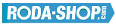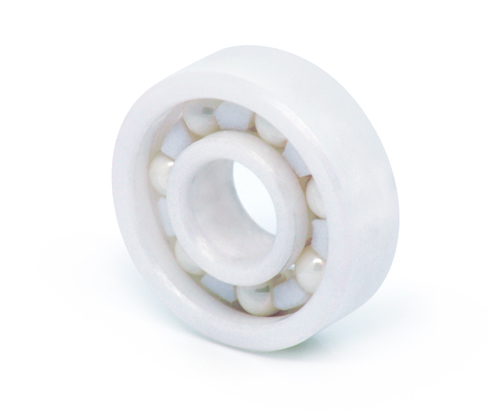# Hybrid and Ceramic BearingsManufacturer Product Image Item Name Price### 6201-CE-SI3N4-PFTE

Solid Ceramic Bearings Ceramic Open(No Shields) Dimension d=12 Dimension D=32 Dimension B=10 Dinamic Load Rating=4080 Static Load Rating=1830 Weight=0.037gr. Speed limit Grease=N/A Speed limit Oil=0 Flanged
inside diameter = 12
outside diameter = 32
width = 10### 6201-CE-ZR02-PFTE

Solid Ceramic Bearings Ceramic Open(No Shields) Dimension d=12 Dimension D=32 Dimension B=10 Dinamic Load Rating=4080 Static Load Rating=1830 Weight=0.037gr. Speed limit Grease=N/A Speed limit Oil=0 Flanged
inside diameter = 12
outside diameter = 32
width = 10
call### 6202-CE-SI3N4-PFTE

Solid Ceramic Bearings Ceramic Open(No Shields) Dimension d=15 Dimension D=35 Dimension B=11 Dinamic Load Rating=4590 Static Load Rating=2250 Weight=0.045gr. Speed limit Grease=N/A Speed limit Oil=0 Flanged
inside diameter = 15
outside diameter = 35
width = 11### 6202-CE-ZR02-PFTE

Solid Ceramic Bearings Ceramic Open(No Shields) Dimension d=15 Dimension D=35 Dimension B=11 Dinamic Load Rating=4590 Static Load Rating=2250 Weight=0.045gr. Speed limit Grease=N/A Speed limit Oil=0 Flanged
inside diameter = 15
outside diameter = 35
width = 11
€101.88### 6203

Solid Ceramic Bearings Ceramic Open(No Shields) Dimension d=17 Dimension D=40 Dimension B=12 Dinamic Load Rating=5730 Static Load Rating=2880 Weight=0.067gr. Speed limit Grease=N/A Speed limit Oil=0
inside diameter =
outside diameter =
width =### 6203-CE-ZR02-PFTE

Solid Ceramic Bearings Ceramic Open(No Shields) Dimension d=17 Dimension D=40 Dimension B=12 Dinamic Load Rating=5730 Static Load Rating=2880 Weight=0.067gr. Speed limit Grease=N/A Speed limit Oil=0 Flanged
inside diameter =
outside diameter =
width =
call### 6204-CE-SI3N4-PFTE

Solid Ceramic Bearings Ceramic Open(No Shields) Dimension d=20 Dimension D=47 Dimension B=14 Dinamic Load Rating=7680 Static Load Rating=3960 Weight=0.107gr. Speed limit Grease=N/A Speed limit Oil=0 Flanged
inside diameter = 20
outside diameter = 47
width = 14### 6204-CE-ZR02-PFTE

Solid Ceramic Bearings Ceramic Open(No Shields) Dimension d=20 Dimension D=47 Dimension B=14 Dinamic Load Rating=7680 Static Load Rating=3960 Weight=0.107gr. Speed limit Grease=N/A Speed limit Oil=0 Flanged
inside diameter = 20
outside diameter = 47
width = 14
call### 6205-CE-SI3N4-PFTE

Solid Ceramic Bearings Ceramic Open(No Shields) Dimension d=25 Dimension D=52 Dimension B=15 Dinamic Load Rating=840 Static Load Rating=4710 Weight=0.129gr. Speed limit Grease=N/A Speed limit Oil=0 Flanged
inside diameter = 25
outside diameter = 52
width = 15### 6205-CE-ZR02-PFTE

Solid Ceramic Bearings Ceramic Open(No Shields) Dimension d=25 Dimension D=52 Dimension B=15 Dinamic Load Rating=840 Static Load Rating=4710 Weight=0.129gr. Speed limit Grease=N/A Speed limit Oil=0 Flanged
inside diameter = 25
outside diameter = 52
width = 15
call